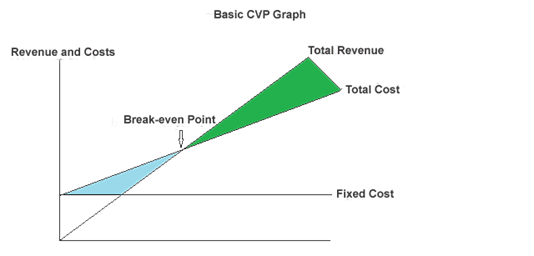1. What do You Mean by Break-Even Point Analysis?

The study of revenues generated and the cost incurred in comparison to the sales volume of any business unit and the analysis of the exact level or point where the sales revenues become equivalent to the costs incurred is known as the Break Even Point Analysis.The study of revenues generated and the cost incurred in comparison to the sales volume of any business unit and the analysis of the exact level or point where the sales revenues become equivalent to the costs incurred is known as the Break Even Point Analysis.

Question

What is Break-even point?

Solution

That particular point or level in a business where the total income or revenues generated from sales become equal to the costs incurred in the business transactions is known as the Break-Even Point. It is at this point where there is a situation of “no profit and no loss”. Although there are no profits generated by the sales, there are no losses as well since the all the costs have been paid by the organization.

Since at the Break-Even point there is a situation of “no profit and no loss”, therefore, the same can be demonstrated by the below equation:

Sales = Variable cost + Fixed costs

Therefore from the above postulation and equation, the below inferences can be drawn:

1.      If the sales revenues exceed the Break-Even Point, it results in the profits for the organization

2.      If the sales revenues decrease than the Break-Even Point, it results in losses for the organization

3.   Example of Break-Even Point

Let us consider a situation where the fixed cost of manufacturing a product is \$500 and the variable cost is \$5 per unit.

If the total sale revenues generated are at the rate of \$10 for 100 units, then the total revenue amounts to \$1000.

Here, the total amount of sales revenue generated (i.e. \$1000) equals the cost of manufacturing of these products (i.e. \$500 + \$500 = \$ 1000).

(Total Cost = Fixed cost + Variable Cost = \$500 + \$5 * 100 units)

Therefore, the company has achieved its Break-Even Point and \$1000 is Break-even point in sales dollars

3. Breakeven GraphHere, the green color indicates the profit and the blue color indicate the loss.

4. The formula of Breakeven Point

Under this method, Break-even Point (B.E.P) can be calculated in the following ways:

1. B.E.P in units

2. B.E.P in dollars

3. B.E.P ratio

Question

B.E.P in units: This is calculated by the following formula

B.E.P  = Fixed Cost/ (Selling Price U- Variable Cost P.U)

= Fixed Cost / Contribution P.U.

 Example of Break-Even Point (in Unit) Selling price (sp) \$20 p.u Variable cost \$12 p.u Fixed costs (FC) \$20,000 Units produced \$8,000

Solution

B.E.P Units = Fixed Cost/ Selling Price U- Variable Cost P.U

= 20000/(20-12) = 2500 Units

Question

B.E.P. in dollars:

(A) When selling price and variable cost are known (per unit):

B.E.P = FC/(1-VC/SP)    Or B.E.P = (FC*SP)/C(PU)

(B) When selling price per unit and variable cost per unit is not known:

B.E.P. = FC*SP / (SP- VC)

 Example of Breakeven Point (In Dollars) Selling Price \$20 P.U Variable Cost \$12 P.U Fixed Costs \$25,000 Units Produced 10,000 UNITS

Solution

Contribution p.u = SP P.U.- VC P.U.

=\$20-\$12 = \$8

B.E.P = (25000*20)/8 = \$62,500.

Question

B.E.P Ratio: This is the ratio between breakeven sales and actual sales made by the business concern. It is calculated with the help of following formula:

B.E.P. = (B.E.P Sales/ Actual Sales)*100

 Example of Break Even Point Ratio Direct materials \$20 Direct labor \$10 Variable O.H \$5 Selling price per unit \$40 Fixed costs \$2500 Units sold in 2012 3000 (UNITS) B.E.P in dollars \$20,000

Solution

Actual sales = 3000*40 = 1, 20,000

 B.E Ratio =( B.E.P in rupees/ Actual Sales)*100

= (200000/120000)*100 =16.67%

Still, you think you have more questions than Post your question to our experts

If you need more help in break-even-point-analysis

## Phoenix## Genius## Vikas